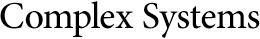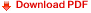## Correlations and Random Information in Cellular AutomataKristian Lindgren
Physical Resource Theory Group, Chalmers University of Technology,
University of Göteborg, S-41296 Göteborg, Sweden

#### Abstract

Infinite one-dimensional cellular automata are studied using information theory. The average information per cell is divided into contributions from different correlation lengths and random variations (measure entropy). It is shown that the measure entropy is non-increasing in time for deterministic rules, and constant for rules which are one-to-one mappings of their first or last argument (almost reversible rules). For probabilistic rules, there is no such general law, but for almost reversible rules where the states are randomly shifted, it is proven that the system evolves towards the maximally disordered state, independent of initial conditions.

It is discussed how some of the information-theoretical concepts are related to analogous concepts in algorithmic information theory, and an equality between algorithmic information and measure entropy is proved.

Numerical and analytical examples are given for specific rules.